Question

Fluid Mechanics

i. (20 points) During a high Reynolds number experiment, the total drag force acting on a spherical body of diameter D = 12 cm subjected to airflow at 1 atm and 5°C is measured to be 5.2 N. The pressure drag acting on the body is calculated by integrating the pressure distribution (measured by the use of pressure sensors throughout the surface) to be 4.9 N. Determine the friction drag coefficient of the sphere, and whether the flow is in turbulence.

i. (20 point) During major windstorms, high vehicles such as RVs and semis maybe thrown off the road and boxcars off their tracks, especially when they are empty and in open areas. Consider a 5000-kg semi that is 9 m long, 2.5 m high,and 2 m wide. The distance between the bottom of the truck and the road is 0.75m. Now the truck is exposed to winds from its side surface. Determine the wind velocity that will tip the truck over to its side, and estimate whether the flow will be in laminar or turbulent regime. Take the air density to be 1.1 kg/m3 and assume the weight to be uniformly distributed. (please refer to Figure P11-43)

i. (20 point) The forming section of a plastics plant puts out a continuous sheet of plastic that is 1.2 m wide and 2 mm thick at a rate of 18 m/min. The sheet is subjected to airflow at a velocity of 4 m/s on both top and bottom surfaces normal to the direction of motion of the sheet. The width of the air cooling section is such that a fixed point on the plastic sheet passes through that section in 2 s. Using properties of air at 1 atm and 60 °C, determine the drag force the air exerts on the plastic sheet in the direction of airflow (please refer to the FigureP11-57).

(20 points) Consider an aircraft that takes off at 190 km/h when it is fully loaded. If the weight of the aircraft is increased by 20% as a result of overloading, determine the speed at which the overloaded aircraft will take off.Verified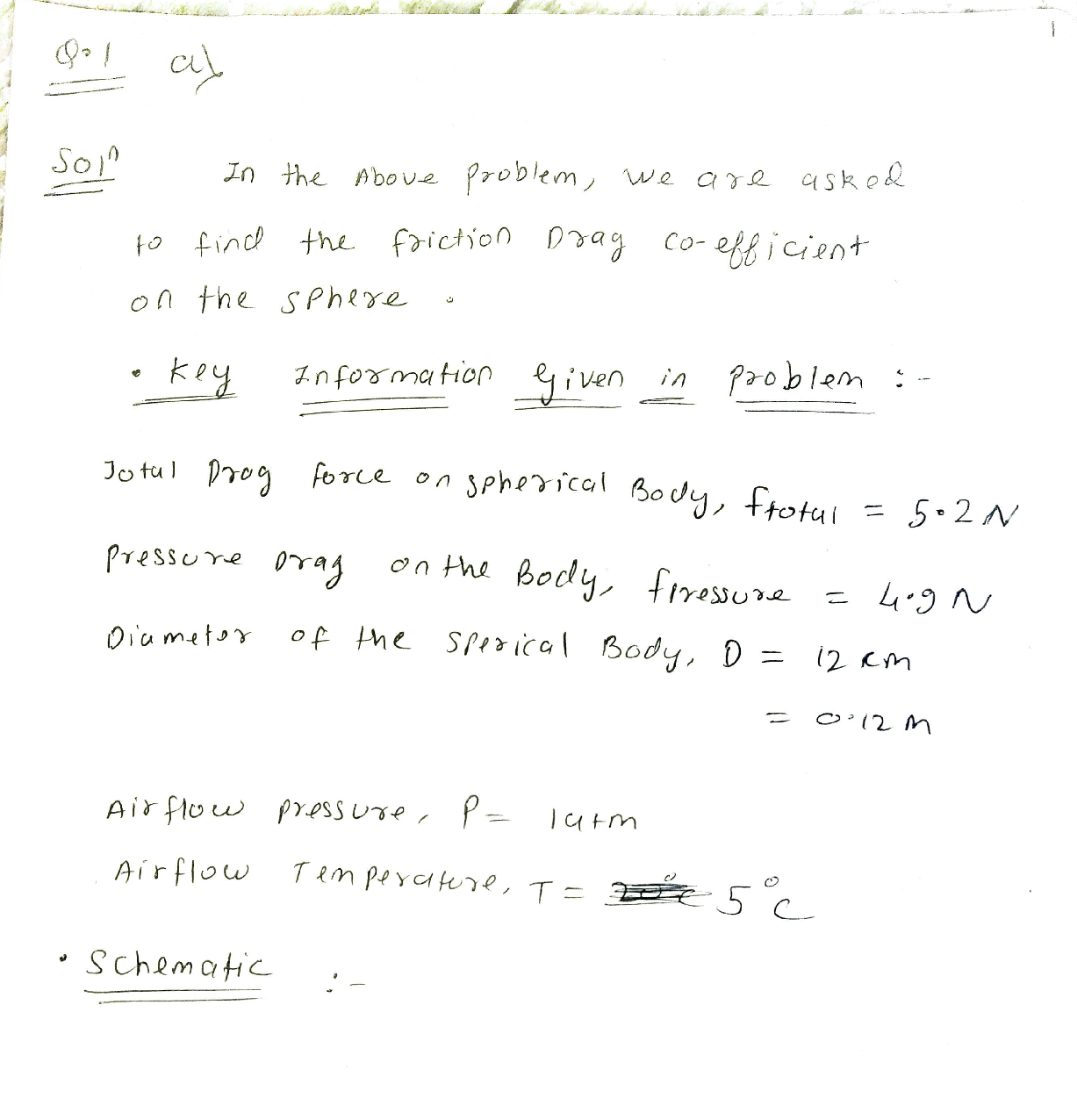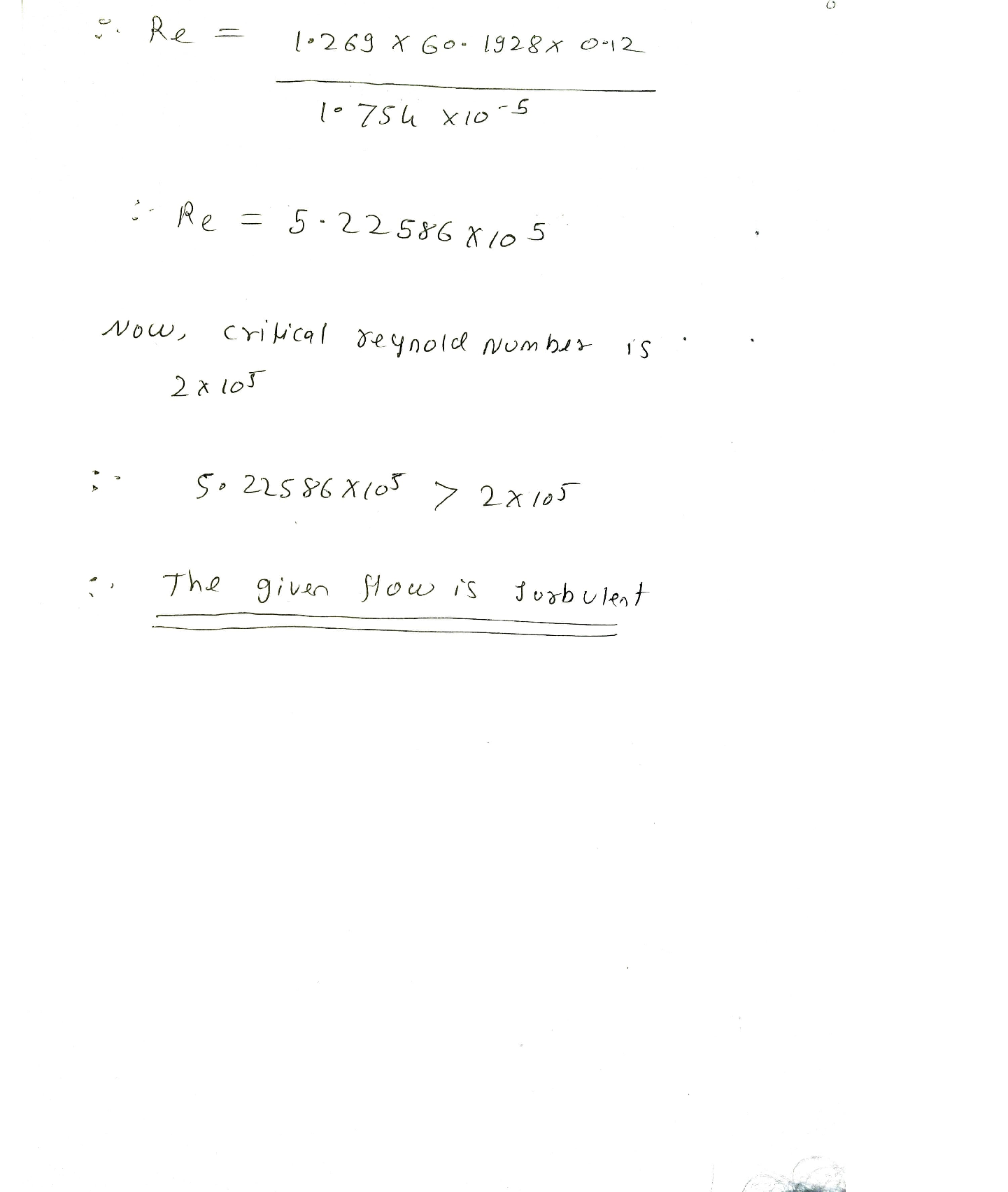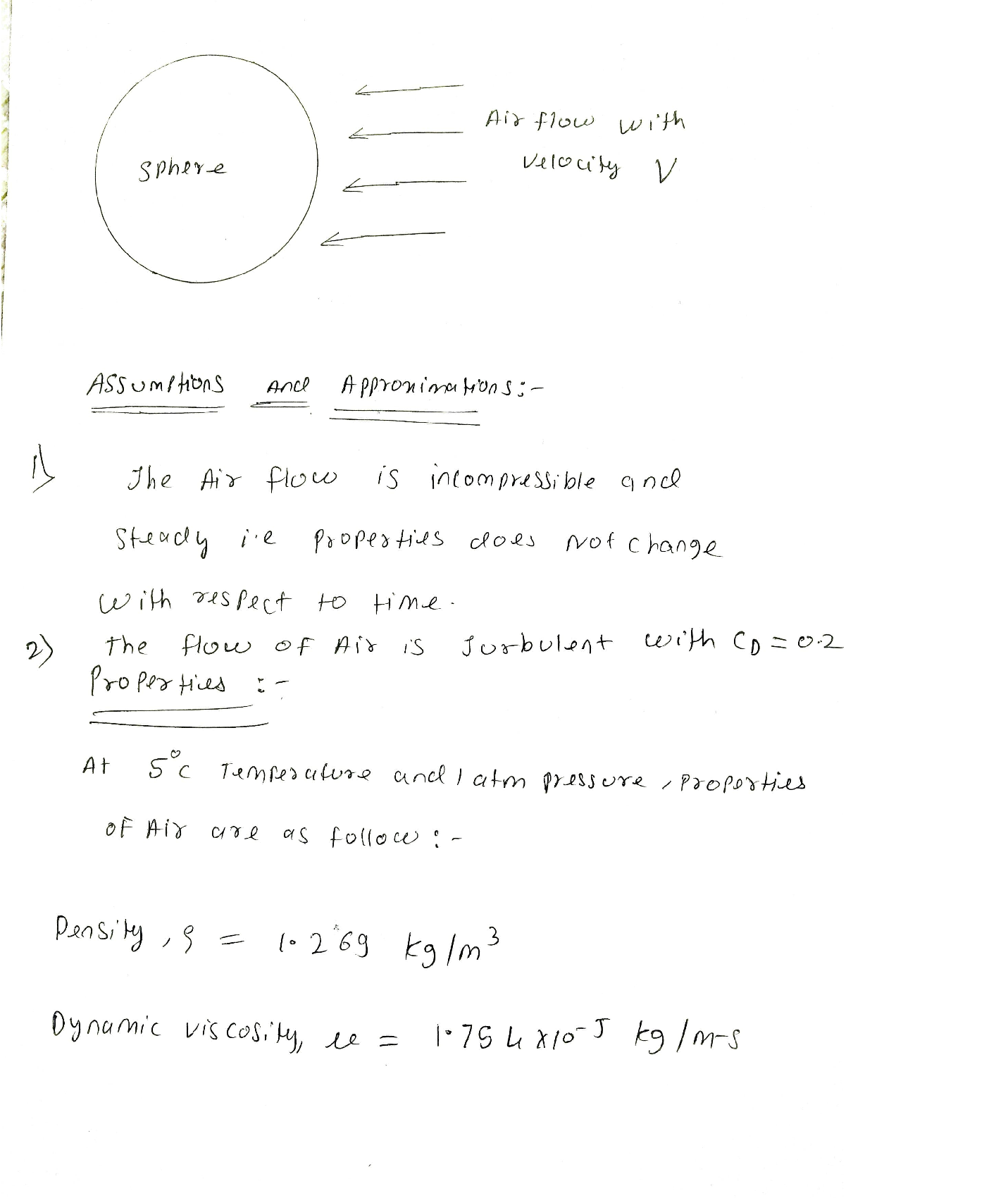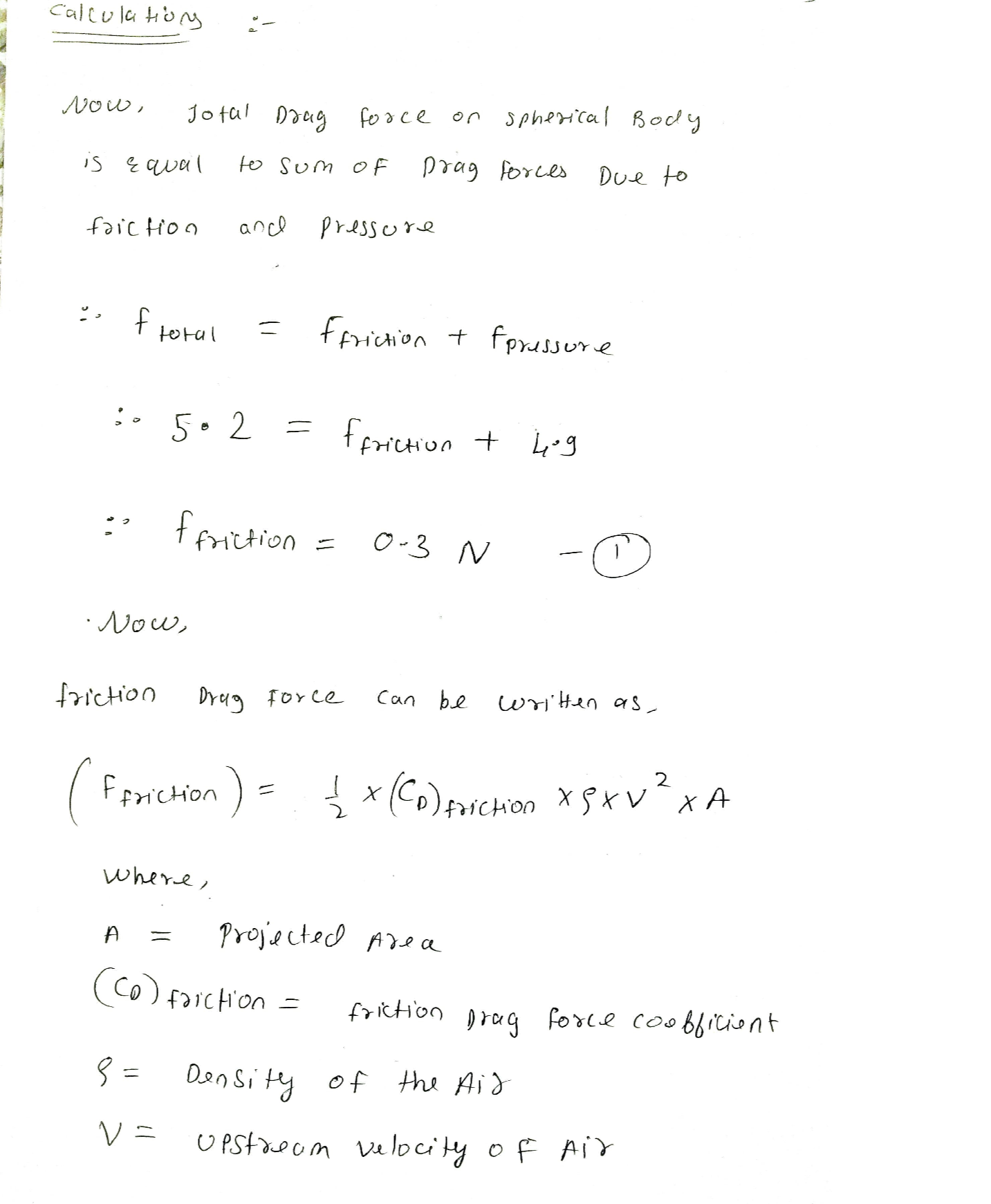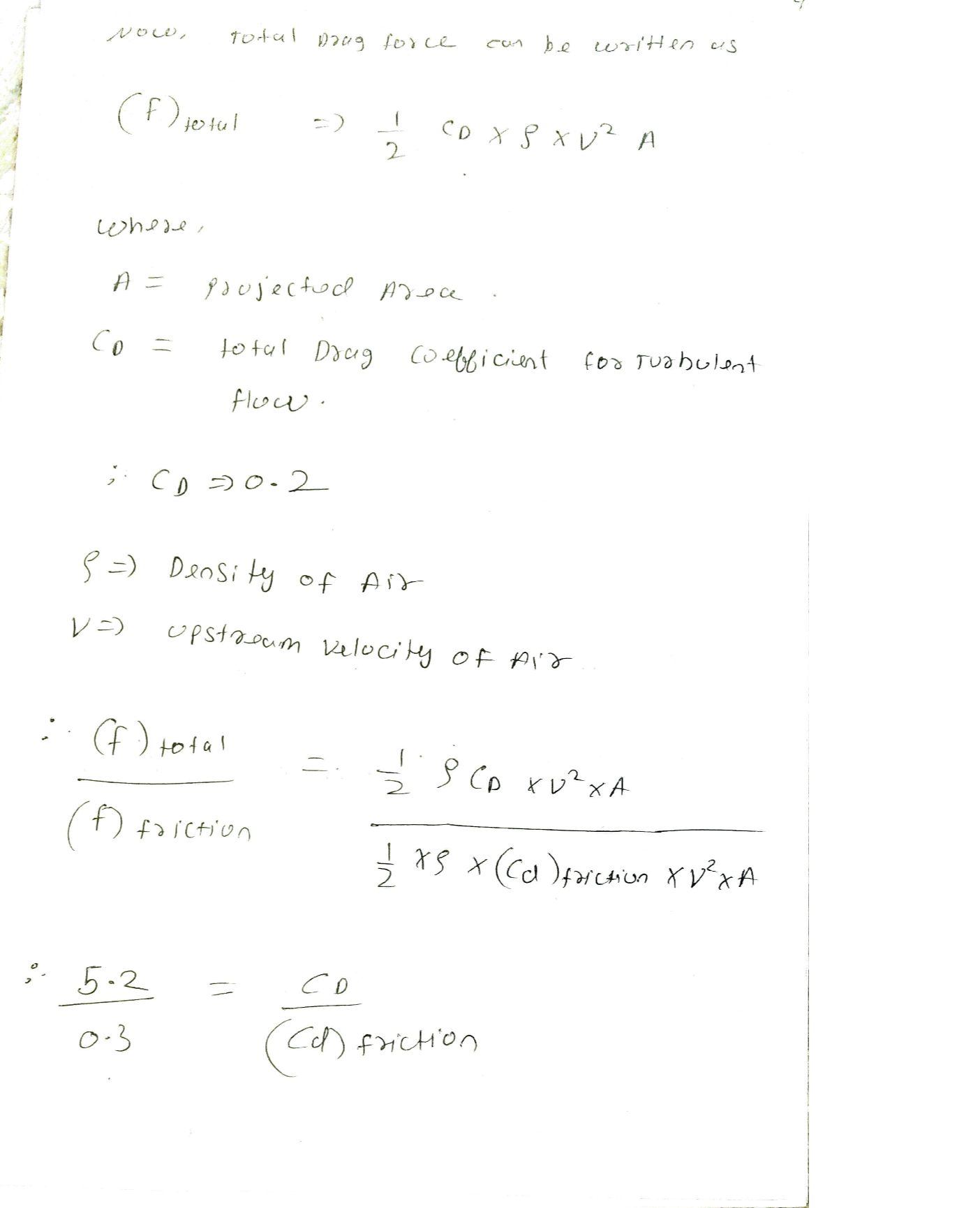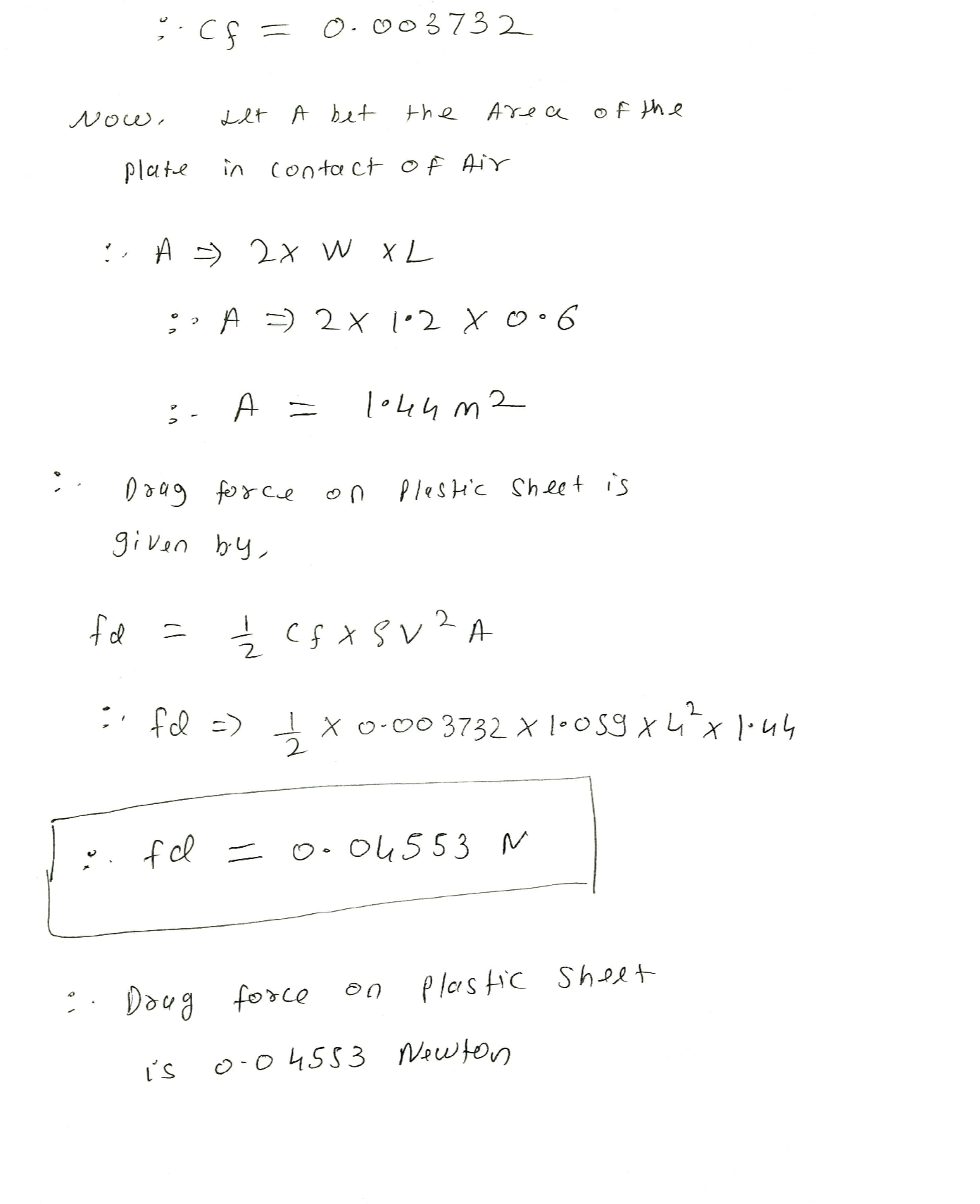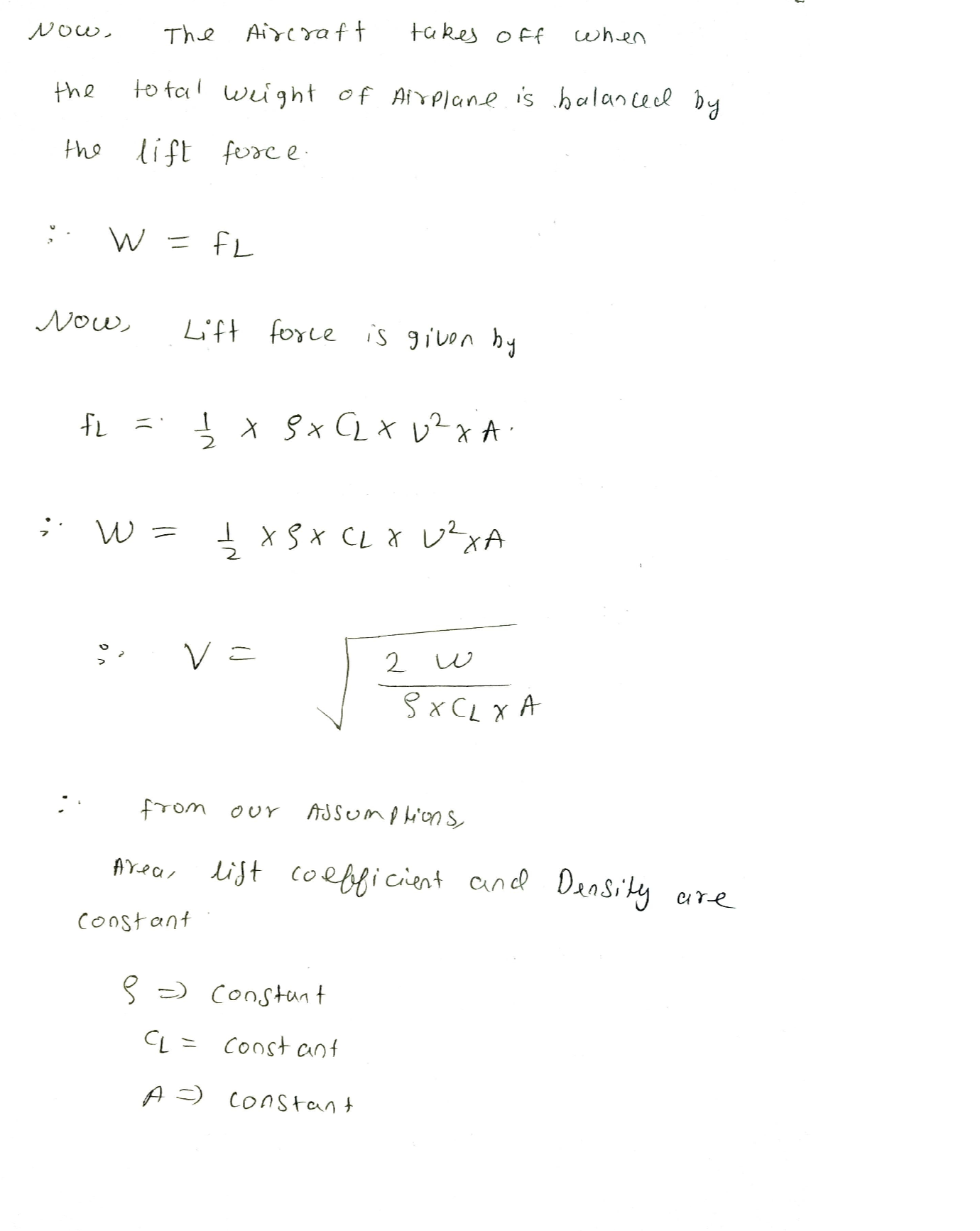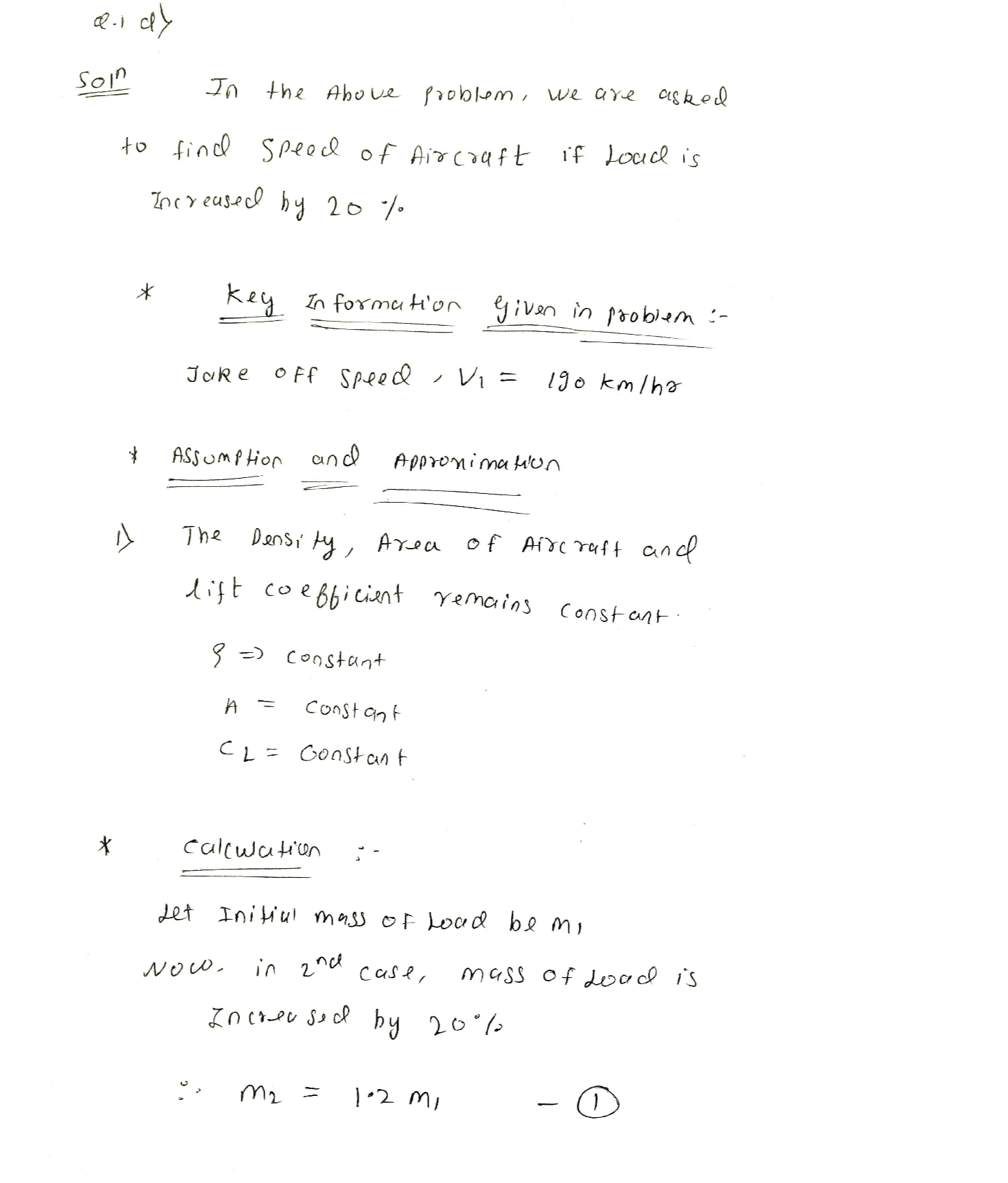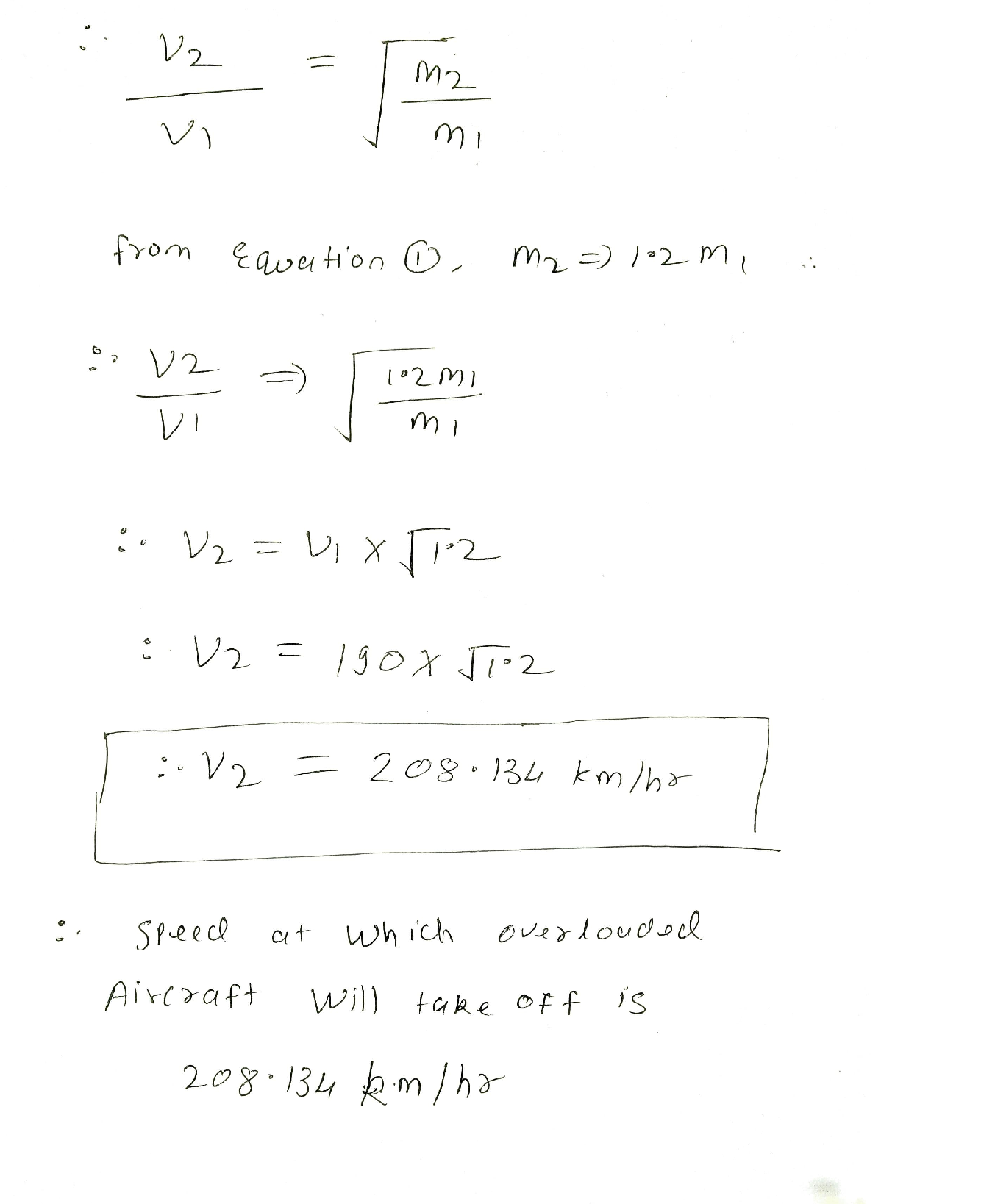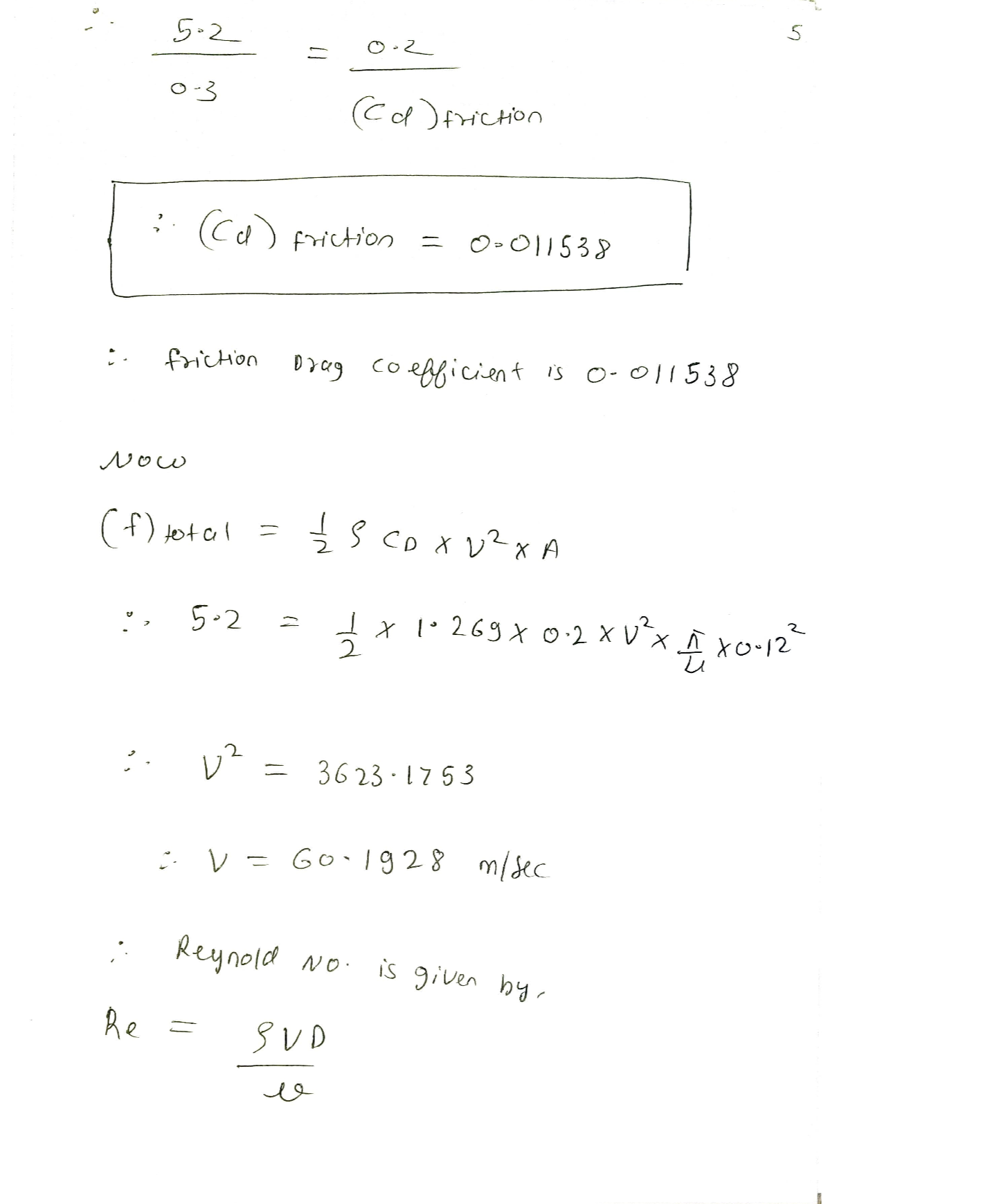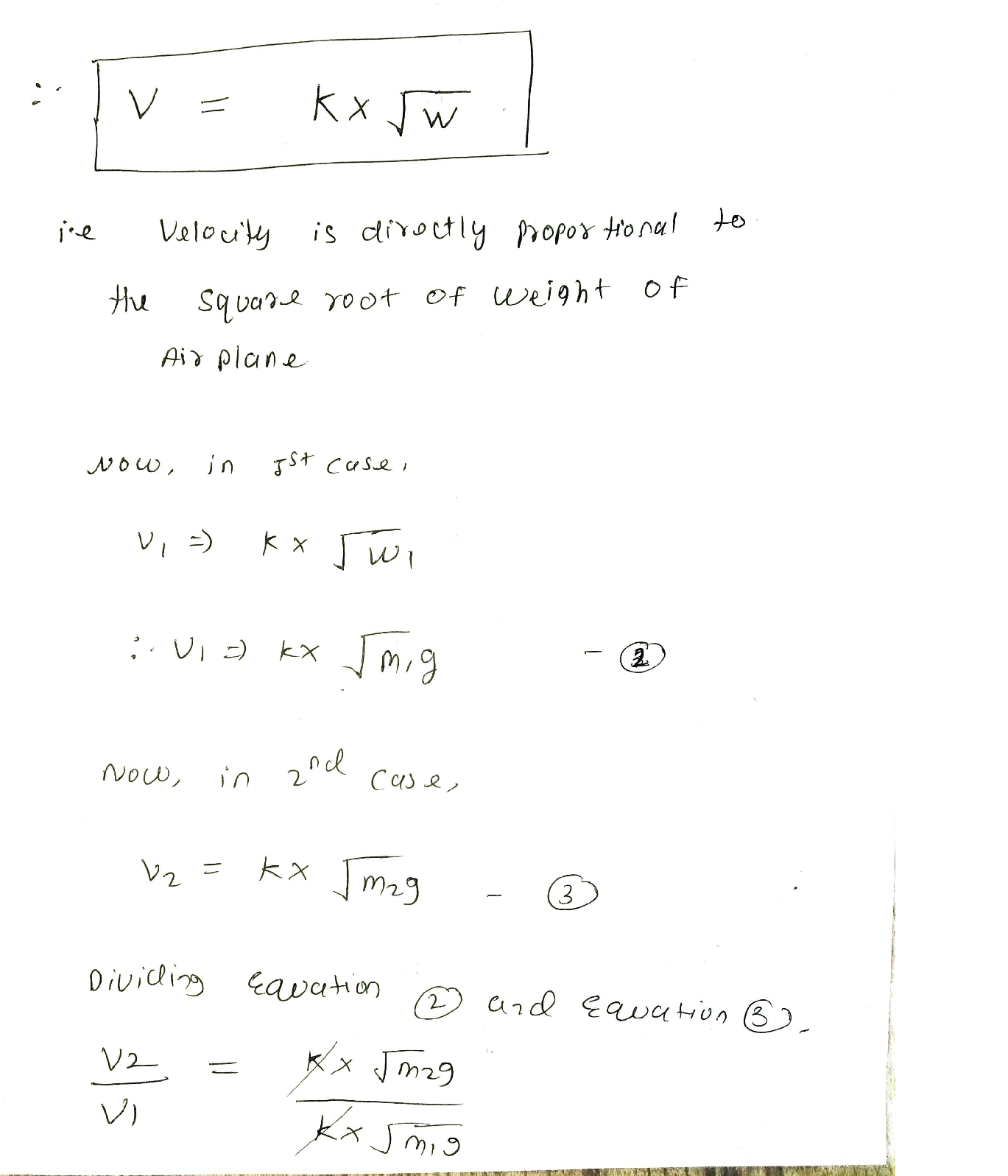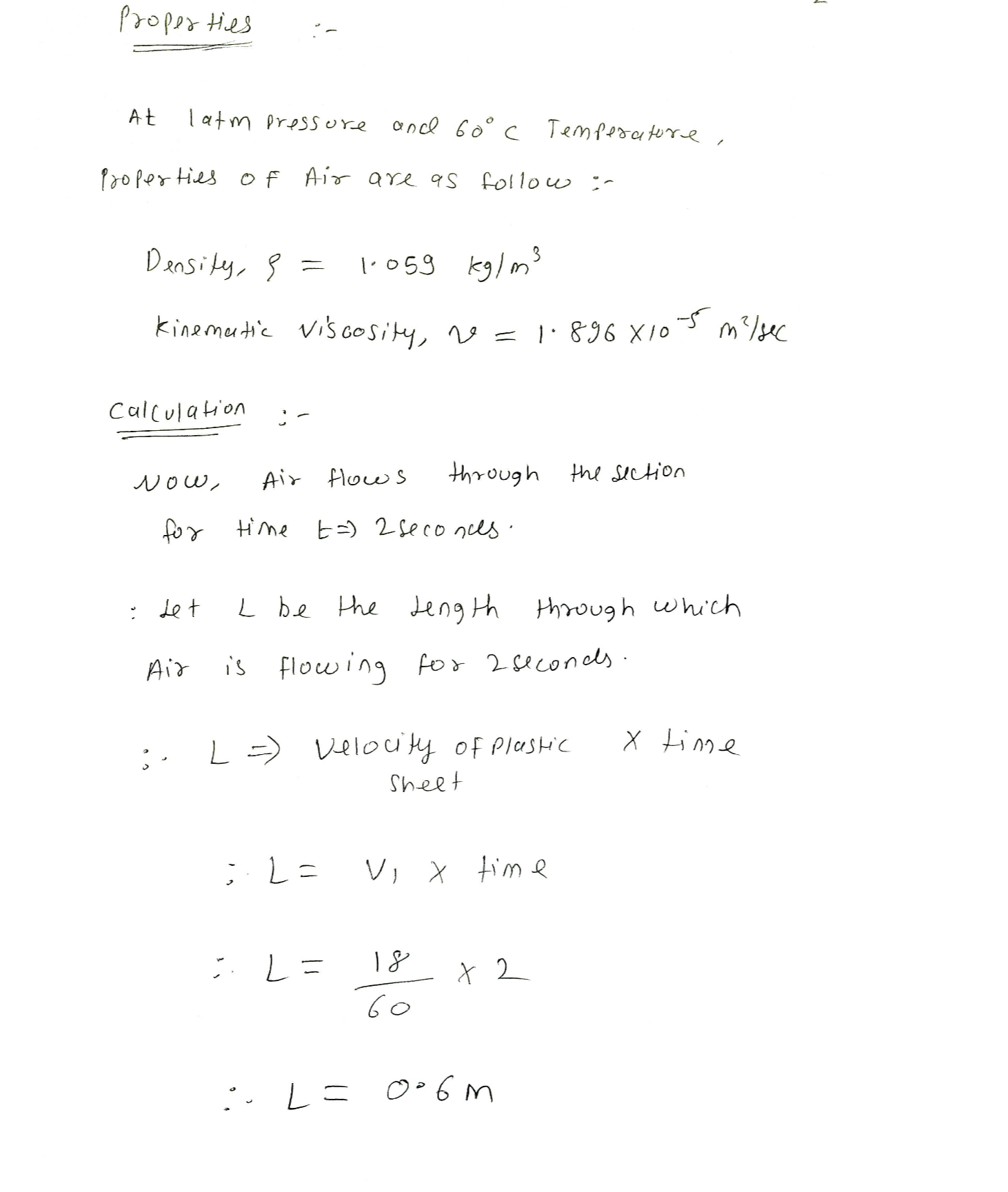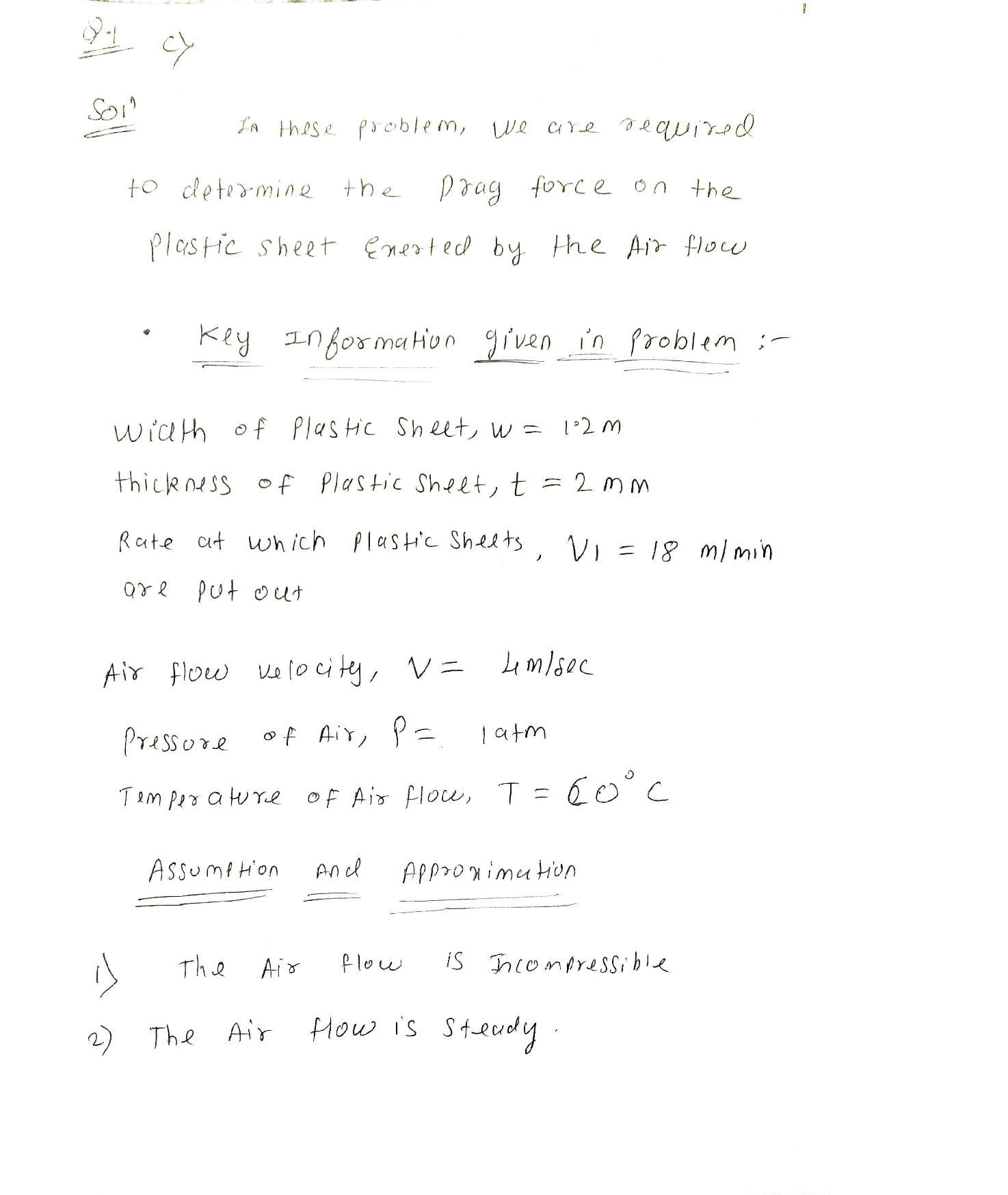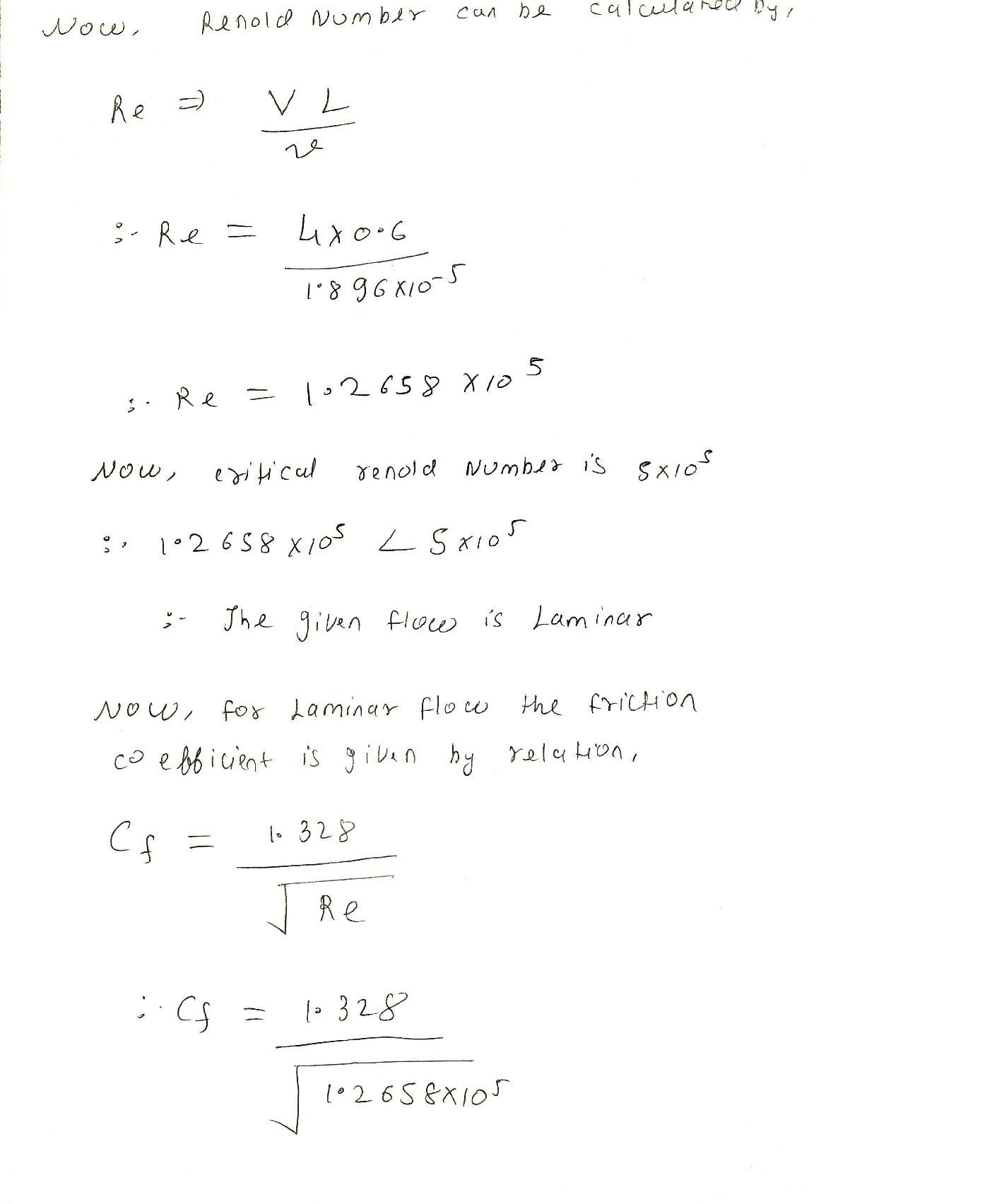### Question 45484Fluid Mechanics

Water in the Belle Fourche Reservoir in Western South Dakotais transported to the water storage reservoir for the town of-Buffalo through a series of three pipes, all with friction factors of 0.02. The first pipe is 500 meters long with a diameter of 0.4meters; the second pipe is 600 meters long with a diameter of0.3 meters; and the third pipe is 500 meters long with a diameter of 0.2 meters. The water surface elevation of the Belle FourcheReservoir is 30 meters higher than the town's storage reservoir.Since the current piping is almost 60 years old, the town of Buffalo has decided to replace the piping system with a single pipe of constant diameter. You have been chosen to calculate the equivalent diameter for the single pipe design and determine the flow rate that will occur. Go to work.

### Question 44899Fluid Mechanics

Transmission lines

### Question 44896Fluid Mechanics

A boat has a volume of 40.0 m³ and a mass of 2.00 tons. What load will push 75.0% of its volume into water? Each Metric ton is 1000 kg.

### Question 44895Fluid Mechanics

A tank has a height of 800 mm and a length of 500 mm. The tank contains a liquid of RD 0.9.Determine:
a. The magnitude of the force on the side of the tank per meter width and its position relative to the base of the tank
b. The force on the base per meter width of tank.

### Question 44894Fluid Mechanics

A closed tank contains three different fluids with a vapor space above the oil, as shown in the figure below. Pressure gauge 1 reads the gauge pressure of the vapor space (P1) and pressure gauge 2 reads the pressure at the bottom of the tank (P2). When P1 = 25 kPa, determine:
a. Height of glycerine (h) in the tank if P2 = 95.66 kPa
. P2 reading if glycerine is replaced with water

### Question 44805Fluid Mechanics

Water flows into cylindrical tank through inlet A and D at rate of 20 ft/s and 5 ft/s respectively. If waterleaves through outlet B and C at rate of 3 ft/s and 10 ft/s respectively.
(A) Derive the continuity equation
\text { Know that } D_{A}=2.5 \text { in, } D_{D}=2 \text { in } D_{B}=3 \text { in and } D_{C}=3.5 \text { in. }
(B) Calculate the change of high h?

### Question 44804Fluid Mechanics

An object which has cubic shape is been floating on the water surface see the diagram below. If the object density is less than water, density find the ratio X/L?

### Question 44803Fluid Mechanics

A force of 15.83 kN is exerted on a piston B (diameter = 250 mm) which fits into a cylinder see the diagram below. How much force is need on piston C to start displacing piston B and Mercury on piston B?

### Question 44802Fluid Mechanics

Given the diagram below if the pressure gauge reads 6.1 psig find Y and X in ft. Given that
\mathrm{SG}_{\mathrm{Aloohal}}=0.9, \mathrm{SG}_{\mathrm{Hg}}=13.6, \text { Alcohol vapor } P=1.7 \mathrm{psig}, Z=4 \mathrm{ft} \text { and } \mathrm{Y}_{\mathrm{H} 2 \mathrm{o}}=62.4 \mathrm{\textrm {lb }} / \mathrm{ft}^{3}

### Question 44801Fluid Mechanics

Given the diagram below, calculate the pressure at A, B, C.
\text { Given that } \mathrm{Y} \text { н2о }=9.79 \mathrm{kN} / \mathrm{m}

### Submit query